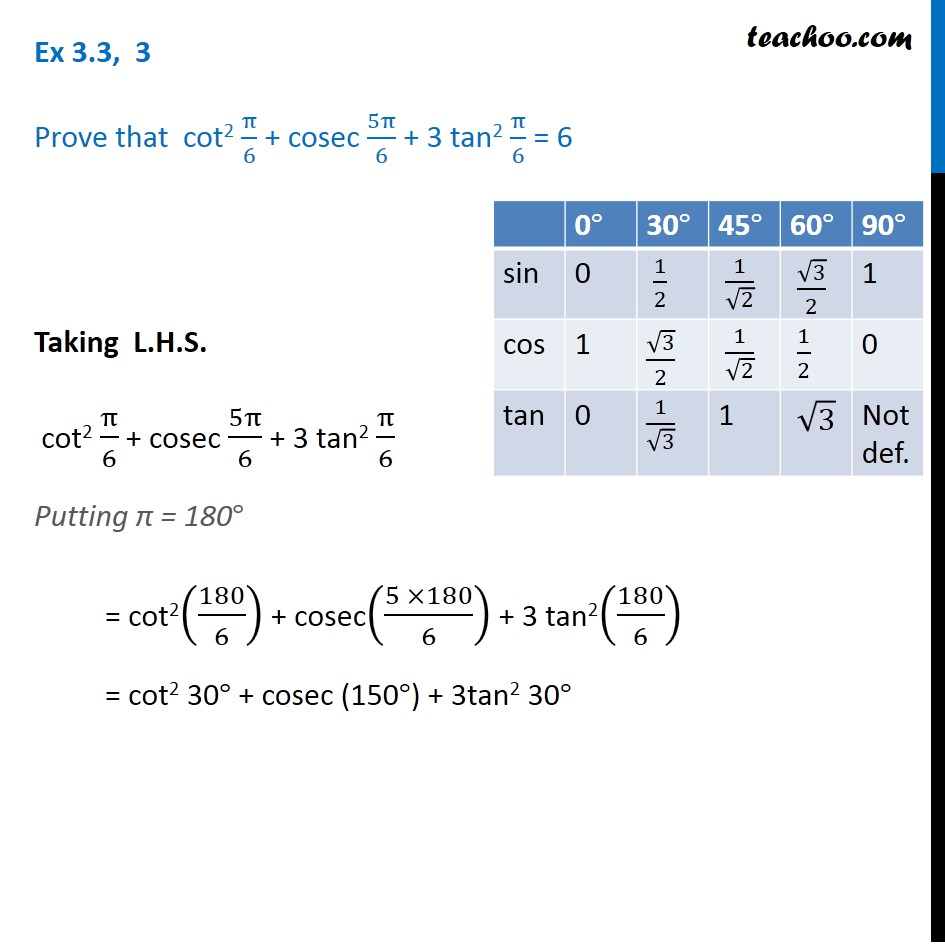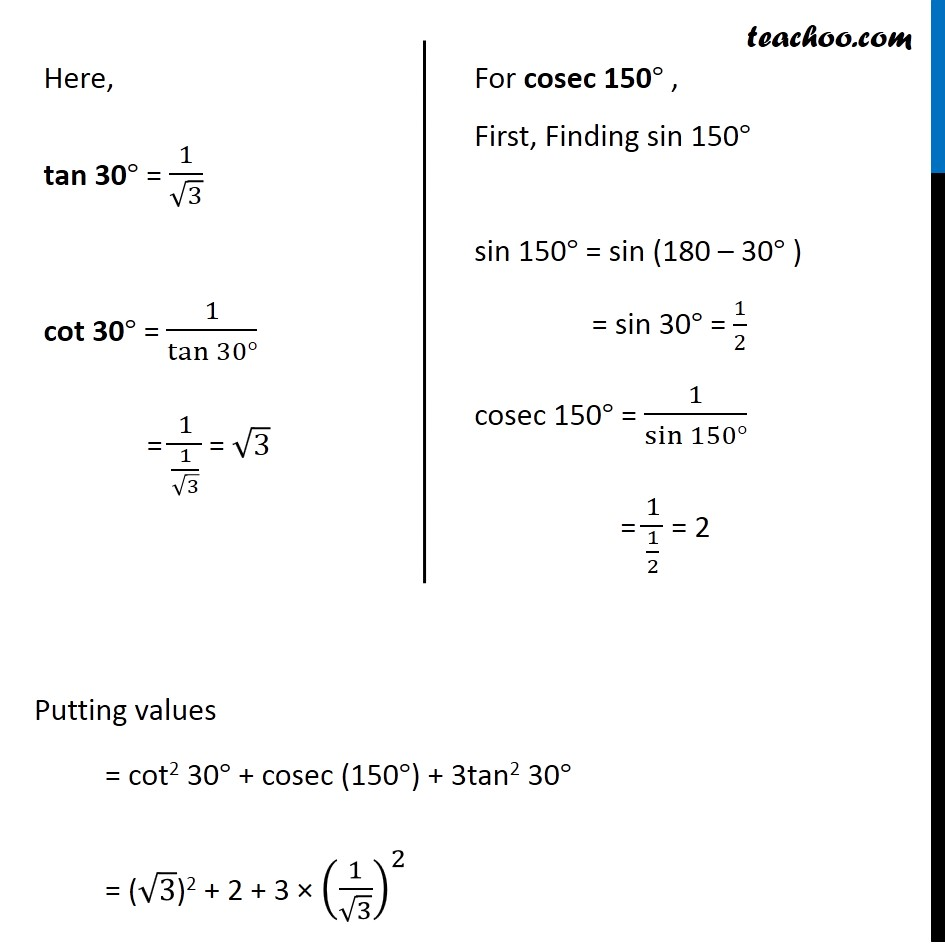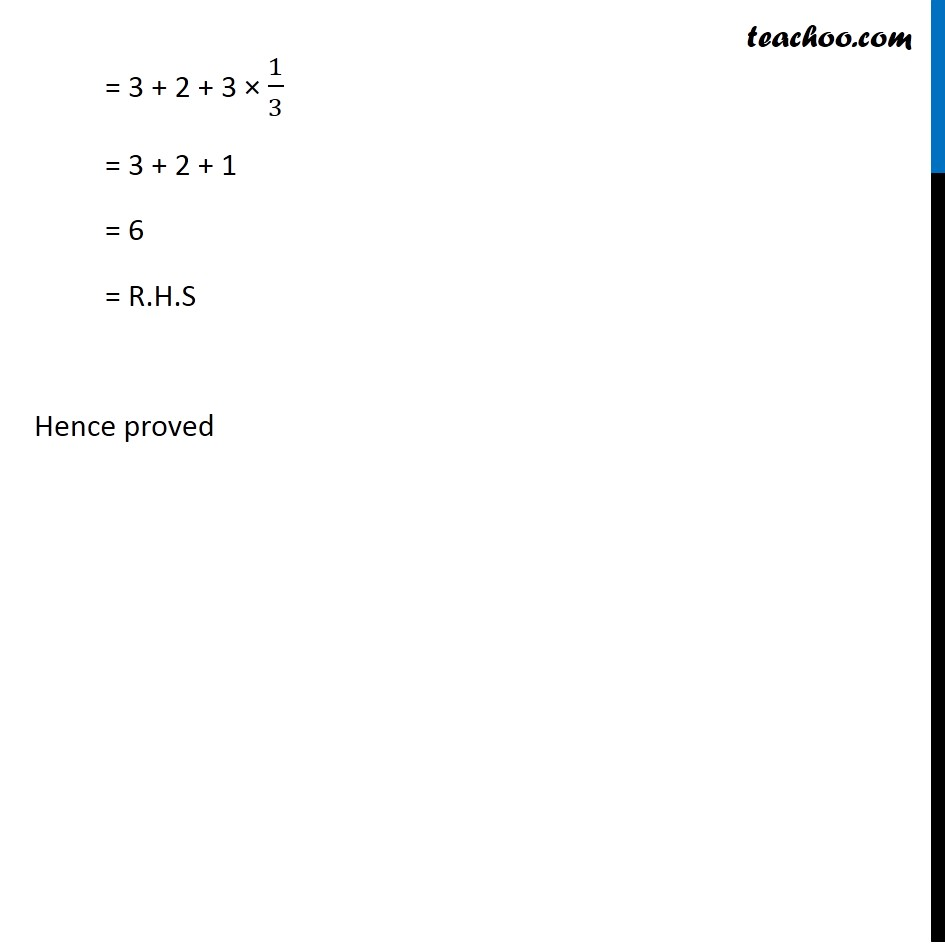Learn All Concepts of Chapter 2 Class 11 Relations and Function - FREE. Check - Trigonometry Class 11 - All Concepts1. Chapter 3 Class 11 Trigonometric Functions
2. Concept wise
3. Finding Value of trignometric functions, given angle

Transcript

Ex 3.3, 3 Prove that cot2 π/6 + cosec 5π/6 + 3 tan2 π/6 = 6 Taking L.H.S. cot2 π/6 + cosec 5π/6 + 3 tan2 π/6 Putting π = 180° = cot2(180/6) + cosec((5 ×180)/6) + 3 tan2(180/6) = cot2 30° + cosec (150°) + 3tan2 30° Here, tan 30° = 1/√3 cot 30° = 1/tan⁡〖30°〗 = 1/(1/√3) = √3 For cosec 150° , First, Finding sin 150° sin 150° = sin (180 – 30° ) = sin 30° = 1/2 cosec 150° = 1/sin⁡〖150°〗 = 1/(1/2) = 2 Putting values = cot2 30° + cosec (150°) + 3tan2 30° = (√3)2 + 2 + 3 × (1/√3)^2 = 3 + 2 + 3 × 1/3 = 3 + 2 + 1 = 6 = R.H.S Hence proved

Finding Value of trignometric functions, given angle

About the AuthorDavneet Singh
Davneet Singh is a graduate from Indian Institute of Technology, Kanpur. He has been teaching from the past 9 years. He provides courses for Maths and Science at Teachoo.